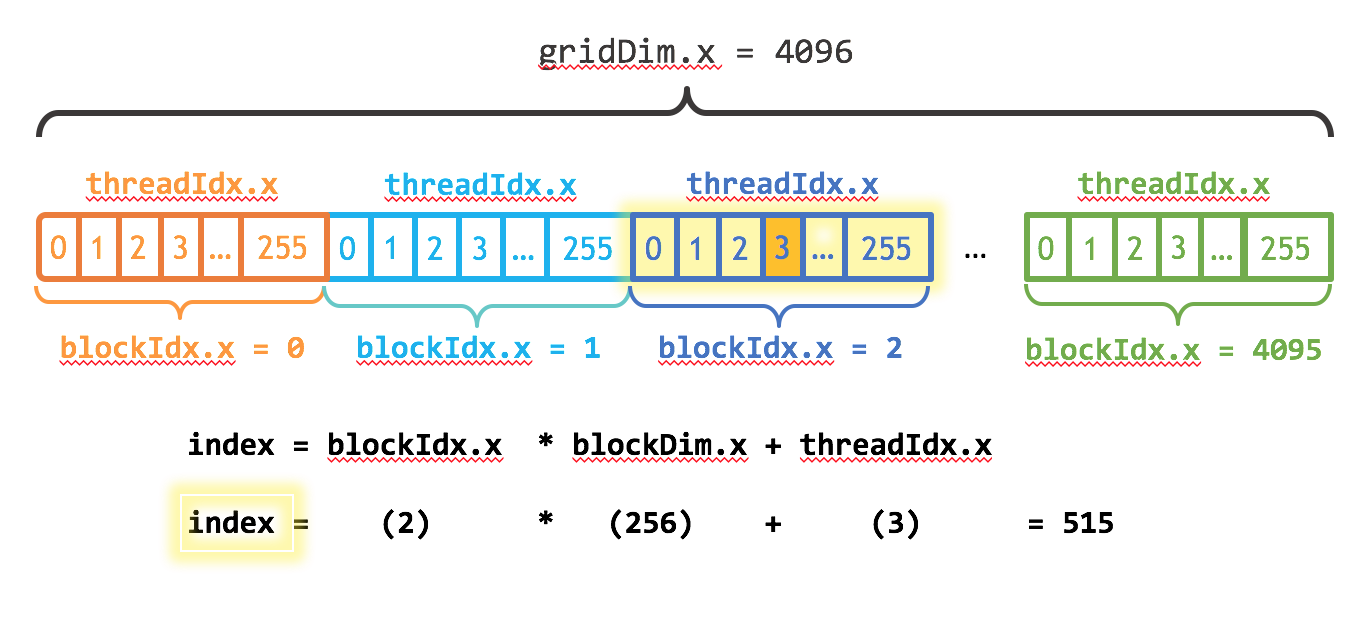# CUDA 的更简单介绍这篇文章是对 CUDA 的一个超级简单的介绍，这是一个流行的并行计算平台和 NVIDIA 的编程模型。我在 2013 年给 CUDA 写了一篇前一篇 “简单介绍” ，这几年来非常流行。但是 CUDA 编程变得越来越简单， GPUs 也变得更快了，所以是时候更新（甚至更容易）介绍了。

CUDA C ++只是使用 CUDA 创建大规模并行应用程序的一种方式。它让您使用强大的 C ++编程语言来开发由数千个并行线程加速的高性能算法 GPUs 。许多开发人员已经用这种方式加速了他们对计算和带宽需求巨大的应用程序，包括支持人工智能正在进行的革命的库和框架 深度学习

## 从简单开始

```#include <iostream>
#include <math.h> // function to add the elements of two arrays
void add(int n, float *x, float *y)
{ for (int i = 0; i < n; i++) y[i] = x[i] + y[i];
} int main(void)
{ int N = 1<<20; // 1M elements float *x = new float[N]; float *y = new float[N]; // initialize x and y arrays on the host for (int i = 0; i < N; i++) { x[i] = 1.0f; y[i] = 2.0f; } // Run kernel on 1M elements on the CPU add(N, x, y); // Check for errors (all values should be 3.0f) float maxError = 0.0f; for (int i = 0; i < N; i++) maxError = fmax(maxError, fabs(y[i]-3.0f)); std::cout << "Max error: " << maxError << std::endl; // Free memory delete [] x; delete [] y; return 0;
}```

`> clang++ add.cpp -o add`

`> ./add Max error: 0.000000`

（在 Windows 上，您可能需要命名可执行文件添加. exe 并使用 `.dd` 运行它。）

```// CUDA Kernel function to add the elements of two arrays on the GPU
__global__
void add(int n, float *x, float *y)
{ for (int i = 0; i < n; i++) y[i] = x[i] + y[i];
}```

## CUDA 中的内存分配

` // Allocate Unified Memory -- accessible from CPU or GPU float *x, *y; cudaMallocManaged(&x, N*sizeof(float)); cudaMallocManaged(&y, N*sizeof(float)); ... // Free memory cudaFree(x); cudaFree(y);`

`add<<<1, 1>>>(N, x, y);`

```#include <iostream>
#include <math.h>
// Kernel function to add the elements of two arrays
__global__
void add(int n, float *x, float *y)
{ for (int i = 0; i < n; i++) y[i] = x[i] + y[i];
} int main(void)
{ int N = 1<<20; float *x, *y; // Allocate Unified Memory – accessible from CPU or GPU cudaMallocManaged(&x, N*sizeof(float)); cudaMallocManaged(&y, N*sizeof(float)); // initialize x and y arrays on the host for (int i = 0; i < N; i++) { x[i] = 1.0f; y[i] = 2.0f; } // Run kernel on 1M elements on the GPU add<<<1, 1>>>(N, x, y); // Wait for GPU to finish before accessing on host cudaDeviceSynchronize(); // Check for errors (all values should be 3.0f) float maxError = 0.0f; for (int i = 0; i < N; i++) maxError = fmax(maxError, fabs(y[i]-3.0f)); std::cout << "Max error: " << maxError << std::endl; // Free memory cudaFree(x); cudaFree(y); return 0;
}```

CUDA 文件具有文件扩展名； `.cu` 。所以把代码保存在一个名为

```> nvcc add.cu -o add_cuda
Max error: 0.000000```

## 介绍一下！

```\$ nvprof ./add_cuda
==3355== NVPROF is profiling process 3355, command: ./add_cuda
Max error: 0
==3355== Profiling result:
Time(%) Time Calls Avg Min Max Name
100.00% 463.25ms 1 463.25ms 463.25ms 463.25ms add(int, float*, float*)
...```

## 把线捡起来

`add<<<1, 256>>>(N, x, y);`

```__global__
void add(int n, float *x, float *y)
{ int index = threadIdx.x; int stride = blockDim.x; for (int i = index; i < n; i += stride) y[i] = x[i] + y[i];
}```

`add` 函数没有太大变化。事实上，将 `index` 设置为 0 ， `stride` 设置为 1 会使其在语义上与第一个版本相同。

```Time(%) Time Calls Avg Min Max Name
100.00% 2.7107ms 1 2.7107ms 2.7107ms 2.7107ms add(int, float*, float*)```

## 走出街区

CUDA GPUs 有许多并行处理器组合成流式多处理器或 SMs 。每个 SM 可以运行多个并发线程块。例如，基于 Tesla 的 Tesla P100 帕斯卡 GPU 体系结构 有 56 个短消息，每个短消息能够支持多达 2048 个活动线程。为了充分利用所有这些线程，我应该用多个线程块启动内核。

```int blockSize = 256;
int numBlocks = (N + blockSize - 1) / blockSize;```__global__
void add(int n, float *x, float *y)
{ int index = blockIdx.x * blockDim.x + threadIdx.x; int stride = blockDim.x * gridDim.x; for (int i = index; i < n; i += stride) y[i] = x[i] + y[i];
}```

```Time(%) Time Calls Avg Min Max Name
100.00% 94.015us 1 94.015us 94.015us 94.015us add(int, float*, float*)```

## 总结

Laptop (GeForce GT 750M) Server (Tesla K80)
Version Time Bandwidth Time Bandwidth
1 CUDA Thread 411ms 30.6 MB/s 463ms 27.2 MB/s
1 CUDA Block 3.2ms 3.9 GB/s 2.7ms 4.7 GB/s
Many CUDA Blocks 0.68ms 18.5 GB/s 0.094ms 134 GB/s

## 练习

1. 浏览 工具包文件 。如果您还没有安装 CUDA ，请查看 快速入门指南 和安装指南。然后浏览 编程指南最佳实践指南 。还有针对各种体系结构的调整指南。
2. 在内核中使用 `printf()` 进行实验。尝试打印出部分或所有线程的 `threadIdx.x``blockIdx.x` 的值。它们是按顺序打印的吗？为什么或者为什么不呢？
3. 在内核中打印 `threadIdx.y``threadIdx.z` （或 `blockIdx.y` ）的值。（同样适用于 `blockDim``gridDim` ）。这些为什么存在？如何让它们采用 0 以外的值（ 1 表示尺寸）？
4. 如果您可以访问 基于 Pascal 的 GPU ，请尝试在其上运行 `add_grid.cu` 。性能比 K80 的结果好还是差？为什么？（提示：阅读关于 Pascal 的 PageMIG 定量引擎和 CUDA 8 统一内存 API 的信息）关于这个问题的详细答案，请参阅 CUDA 初学者的统一内存 一文。